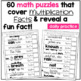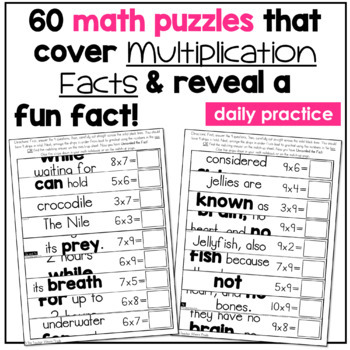# Multiplication Facts Games | 3rd Grade Math Centers | Unravel the FactSubject
Resource Type
File Type
PDF (14 MB|74 pages)
Standards
\$4.00
• Product Description
• Standards

©Unlock the Fact Math Puzzles are an original idea by The Teacher Wears Prada...in a NUTSHELL...students answer the problems, put the puzzle together, which then reveals an INTERESTING FACT! SAY IT WITH ME...CONTENT INTEGRATION!! :)

::Here are 48 MULTIPLICATION Math Puzzles that reveal interesting and fun facts about various animals, the human body, planets/solar system, etc.

These cross-curricular math puzzles are super LOW PREP which I LOVE and NEED. I simply print these out on colored paper, laminate, THEN cut along the lines into strips. It’s so much easier to laminate them first, and cut them out second. Next, I rubber band or bind each set together using a mini binder clip. Once I’m ready to place these out for the students to use during math rotations, I’ll just place them in a small basket.

This resource includes 40 different puzzles PLUS 8 BONUS PUZZLES that cover the following 3rd Grade Multiplication Skills {Common Core}:

***The Common Core standard is printed in the upper left corner of each puzzle.

• Arrays ( 4 puzzles)
• Equal Groups (2 puzzles)
• Groups of/ Multiplication Facts (4 puzzles)
• Fact Families/Related Facts (3 puzzles)
• Multiplication Facts 1-12 (12 puzzles)
• 2s and 10s
• 5s and 10s
• 2s 5s and 10s
• 9s and 11s
• 3s and 4s
• 6s and 7s
• 8s and 12s
• Mixed Multiplication Facts ( 4 puzzles)
• Multiplication Patterns Input Tables--2 puzzles)
• Multiplication Word Problems (2 puzzles)
• Missing Factor (6 puzzles)

::HOW TO USE THIS RESOURCE::

First, the students answer all 9 questions. Using a thin dry erase marker, students will write the answer in the rectangular box. Once they’ve answered all 9 questions, they will put the puzzle strips in order from LEAST to GREATEST. {See example in the PREVIEW} If they have answered the problems correctly and have placed the numbers in order correctly, a FUN FACT will be revealed.

{LOVE being able to tie in so many skills at once!} I hold my students ACCOUNTABLE for everything so I have them record their answers on the recording sheet. Once they’ve checked their answers with the answer key (provided in the file). {I laminate all the answer keys and place them on a ring for students to access easily} I have my students glue their recording sheet in the “Centers” section of their math journal.

You could also just send it home once it’s been checked/graded. I also have my students keep track of the facts they reveal {sneaking in a little writing!}

You can either have them write their facts in their math journal/writing journal/any journal of your choice or use the booklet I’ve provide in this file. I love using the booklet.

OR you don’t need to have your students write their fact at all—it’s totally up to you. I hope you like this resource! I will be making an Unlock the Fact Math Puzzle set for each month and 3rd grade standard.

______________________________

Other Multiplication Resources:

______________________________

Other Unlock the Fact Resources:

3rd Grade Unlock the Fact Math Puzzles: Place Value

3rd Grade Spiral Math Growing Bundle

2nd Grade Spiral Math Growing Bundle

©Unlock the Fact Math Puzzles are an original idea from The Teacher Wears Prada

Fluently multiply and divide within 100, using strategies such as the relationship between multiplication and division (e.g., knowing that 8 × 5 = 40, one knows 40 ÷ 5 = 8) or properties of operations. By the end of Grade 3, know from memory all products of two one-digit numbers.
Determine the unknown whole number in a multiplication or division equation relating three whole numbers. For example, determine the unknown number that makes the equation true in each of the equations 8 × ? = 48, 5 = __ ÷ 3, 6 × 6 = ?.
Use multiplication and division within 100 to solve word problems in situations involving equal groups, arrays, and measurement quantities, e.g., by using drawings and equations with a symbol for the unknown number to represent the problem.
Interpret products of whole numbers, e.g., interpret 5 × 7 as the total number of objects in 5 groups of 7 objects each. For example, describe a context in which a total number of objects can be expressed as 5 × 7.
Total Pages
74 pages